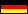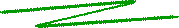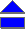The Euler totient function φThe Euler function, or totient function φ is a very important number theoretic function having a deep relationship to prime numbers and the so-called order of integers.

The Euler function φ: NN is a mapping associating to each positive integer n the number φ(n) of integers m n relatively prime to n. (In other words: φ(n) is the number of positive integers m n with gcd(m, n) = 1.) E.g., φ(6) = 2 (since only 1 und 5 are relatively prime to 6), or φ(15) = 8 (for 1, 2, 4, 7, 8, 11, 13, and 14 are relatively prime to 15). The following table shows the function values for the first natural numbers.

 n φ(n) 1 2 3 4 5 6 7 8 9 10 11 12 13 14 15 1 1 2 2 4 2 6 4 6 4 10 4 12 6 8

Do you discover some formation rule? If so, you should go on researching, because you could solve number theoretic problems being open so far... If not, you might be interested in some of the most important properties of the Euler function. The first one is that there exists a formula to compute φ(n): If the prime factorization of n is given by n = p1a1 ... pkak, we have

 φ(n) = n (1 - 1/p1) ... (1 - 1/pk).

Example: 12 = 22 · 3, i.e. φ(12) = 12 · (1 - 1/2) · (1 - 1/3) = 12 · 2 / (2 · 3) = 4. A further property of the totient function is the sum formula: The sum over the Euler function values φ(d) of all divisors d of an integer number n exactly gives n. E.g. for n=12:

φ(1) + φ(2) + φ(3) + φ(4) + φ(6) + φ(12) = 1 + 1 + 2 + 2 + 2 + 4 = 12.

Moreover, the order ordn(m) of an integer m modulo n always divides φ(n). Closely related to the Euler function is the Carmichael function λ.

### References

 © de Vries 2002